• 赋值运算符重载
赋值运算符重载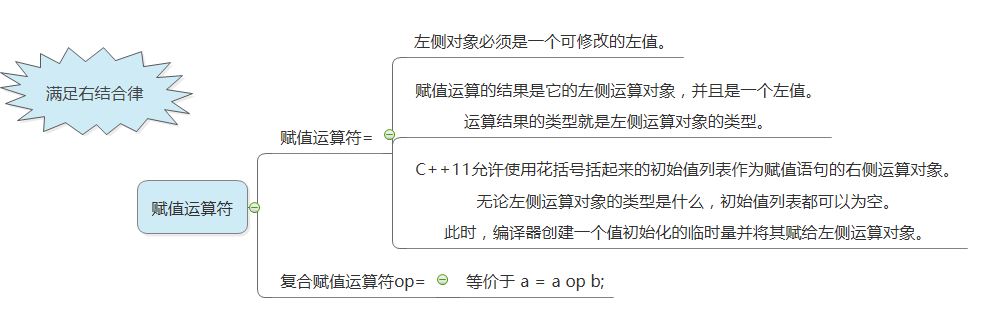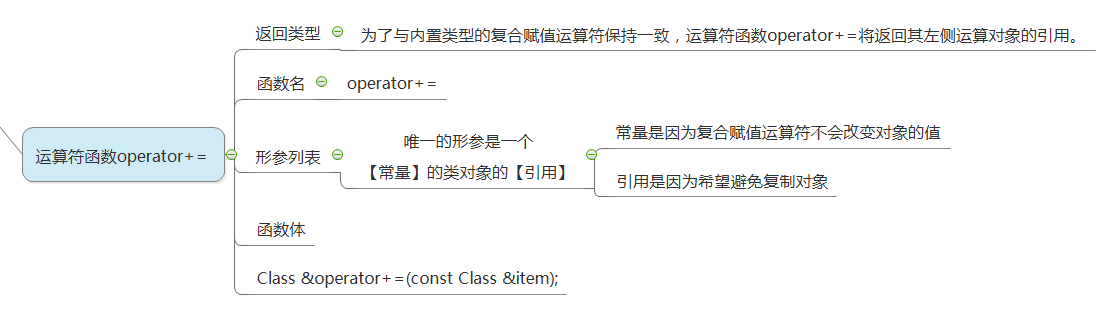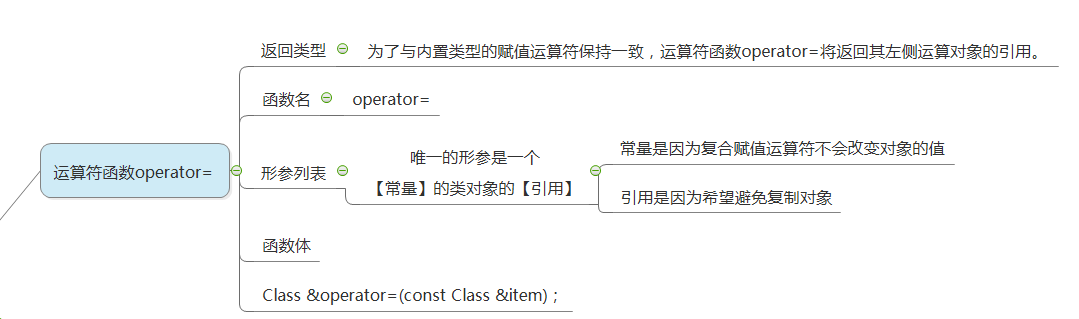类的声明

//
// Created by Rdw on 2017/3/9.
//

#ifndef PROJECT5_TIME_H
#define PROJECT5_TIME_H

#include <ostream>
#include <istream>
using namespace std;

class Time {
private:
int hour;
int minute;
public:
Time();//默认构造函数
Time(int h , int m);//构造函数
~Time();//析构函数

friend ostream &operator<<(ostream &os , const Time &object);

/*重载赋值运算符*/
Time &operator=(const Time&object);
Time &operator+=(const Time&object);
};

#endif //PROJECT5_TIME_H

类的定义

//
// Created by Rdw on 2017/3/9.
//

#include "Time.h"

Time::Time() {

}

Time::Time(int h, int m) {
hour = h;
minute = m;
}

Time::~Time() {

}

Time& Time::operator=(const Time &object) {
hour = object.hour;
minute = object.minute;
return *this;
}

Time& Time::operator+=(const Time &object) {
hour += object.hour;
minute += object.minute;
return *this;
}

ostream& operator<<(ostream &os, const Time &object) {
os << object.hour << "hour " << object.minute << "minutes" ;
os << endl;
return os;
}

类的实现

#include <iostream>
#include "Time.h"

using namespace std;

int main() {
Time time1 = Time(19,22);
cout << time1;

Time time11 = Time(22,22);
cout << time11;

Time time2 = time1;
cout << time2;

time2 += time11;
cout << time2;
}



测试结果

E:\Project5\cmake-build-debug\Project5.exe
19hour 22minutes
22hour 22minutes
19hour 22minutes
41hour 44minutes

Process finished with exit code 0 
展开全文C++ c++
• 赋值运算符重载说明语法特性自实现存在的问题重析构内存泄漏自赋值解决 赋值运算符重载 说明 赋值运算符重载是用一个已有对象，给另一个对象赋值，两个对象均已创建结束后，发生赋值行为。 语法 格式固定 类名 { ...
 赋值运算符重载说明语法特性自实现存在的问题重析构内存泄漏自赋值解决小结
赋值运算符重载
说明
赋值运算符重载是用一个已经存在的对象，给另一个已经存在的对象赋值，两个对象均已创建结束后，发生赋值行为。
语法
格式固定
类名
{
类名 & operator=(const 类名 & 源对象)
拷贝体
}

class A
{
A & operator=(const A & another)
{
//函数体
return *this;
}
};

特性
#include <iostream>
using namespace std;

struct Data
{
public:
Data(int y = 2016, int m = 6, int d = 6)
:year(y), month(m), day(d), space(new char)
{}

void dis()
{
cout << " year " << year << " month " << month << " day " << day << endl;
}
private:
int year;
int month;
int day;
char* space;
};

int main()
{
Data d(2018, 8, 8);
d.dis();
Data d2(2019, 9, 9);
d2 = d;
d2.dis();
return 0;
}

我们可以看到两个打印出来是一样的：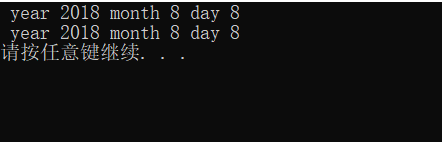我们可以看到我们并没有自己写赋值运算符重载，但是我们确实赋值成功了，并且正确赋值，我们在这里进行说明。
系统默认提供的赋值运算符重载。也是一种浅赋值行为。
我们要理解一下拷贝构造器和赋值运算符重载的区别，拷贝构造器是原来不存在直接进行复制一份，赋值运算符重载是本来就有两份，然后拿第一份的将第二份覆盖。
如果对象中不存在堆内存空间，此时系统默认提供的赋值运算符重载可以满足需求。如果对象中含有堆上的内存空间则需要自实现完成深拷贝。
格式语法固定。
一旦自实现，系统默认提供的赋值运算符重载将不存在。
如果我们实现了但是里面没有内容，那么就不能实现赋值运算符重载：
#include <iostream>
using namespace std;

struct Data
{
public:
Data(int y = 2016, int m = 6, int d = 6)
:year(y), month(m), day(d), space(new char)
{}
Data& operator = (const Data& another)
{
}
void dis()
{
cout << " year " << year << " month " << month << " day " << day << endl;
}
private:
int year;
int month;
int day;
char* space;
};

int main()
{
Data d(2018, 8, 8);
d.dis();
Data d2(2019, 9, 9);
d2 = d;
d2.dis();
return 0;
}

运行结果为：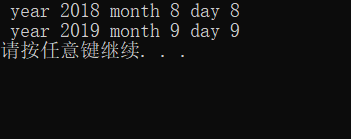我们可以看到赋值运算符没有重载成功。
那么我们自实现运算符重载：
#include <iostream>
using namespace std;

struct Data
{
public:
Data(int y = 2016, int m = 6, int d = 6)
:year(y), month(m), day(d), space(new char)
{}
Data& operator = (const Data& another)
{
this->year = another.year;
this->month = another.month;
this->day = another.day;
return *this;
}
void dis()
{
cout << " year " << year << " month " << month << " day " << day << endl;
}
private:
int year;
int month;
int day;
char* space;
};

int main()
{
Data d(2018, 8, 8);
d.dis();
Data d2(2019, 9, 9);
d2 = d;
d2.dis();
return 0;
}

运行结果为：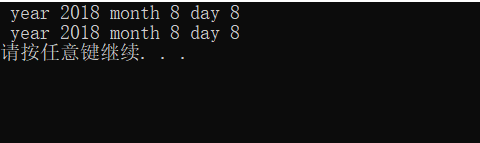我们可以看到上面实例中实现的赋值运算符重载和系统默认提供的赋值运算符重载实现的结果是一样的。
我们来说明一下，为什么在运算符重里面加入了return  * this：
我们只给出main函数中的代码，其他代码不变：
int main()
{
Data d(2018, 8, 8);
d.dis();
Data d2(2019, 9, 9);
d2 = d;
d2.dis();
//使用返回this指针
Data d3;
d3 = d2 = d;     //与下面一行代码实现意义完全不相同
d3.operator=(d2.operator=(d));
return 0;
}

上面的意思就是说：d赋值给d2，整个表达式返回了d2，然后d2作为参数进行传递，然后将d2赋值给d3，整个表达式又返回d3。
自实现存在的问题
重析构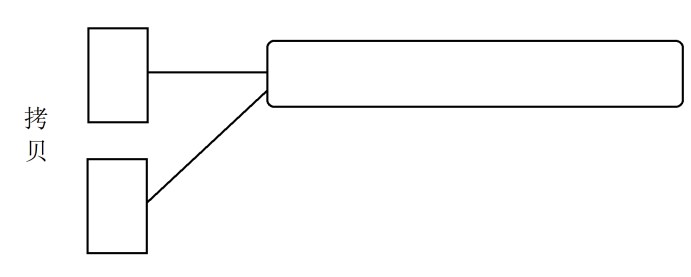那么在释放内存的时候就会出现重析构问题。
内存泄漏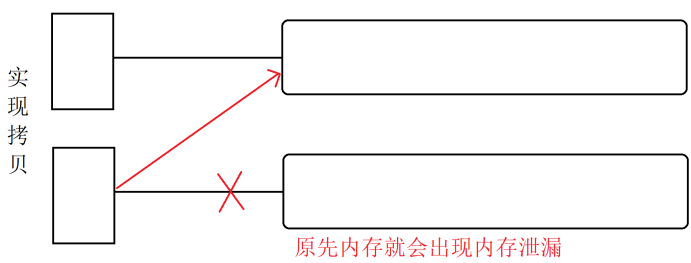解决：先把自己之前的内存进行释放，然后进行深拷贝。
自赋值
自赋值意思就是说当出现自己赋值给自己的时候，那么原来的内存空间被释放了，但是原来的内存空间里面还是自己本身的，如果释放之后就无法进行赋值运算符重载，所以我们在自实现的是时候就要解决这个问题。
解决
代码实现：
#define _CRT_SECURE_NO_WARNINGS
#include <string.h>
#include <iostream>
using namespace std;

struct Data
{
public:
Data(int y = 2016, int m = 6, int d = 6)
:year(y), month(m), day(d), space(new char)
{}

Data& operator = (const Data& another)//赋值运算符重载
{
if (this == &another)   //解决自赋值的问题
return *this;         //实现链式表达
else
{
delete[]this->space;    //解决内存泄漏的问题
space = new char[strlen(another.space) + 1];
strcpy(space, another.space);
}
year = another.year;
month = another.month;
day = another.day;
}

~Data()        //解决重析构的问题
{
cout << "~~~~~~~~" << this << endl;  //指向当前对象的指针
delete[]space;
}

void dis()
{
cout << " year " << year << " month "
<< month << " day " << day << endl;
}
private:
int year;
int month;
int day;
char* space;
};

int main()
{
Data d(2018, 8, 8);
d.dis();
Data d2(2019, 9, 9);
d2 = d;
d2.dis();
return 0;
}

上面自实现代码就解决了这三个问题。
运行结果：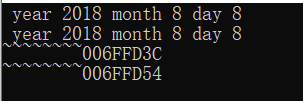接下来进行说明我们在：
Data & operator = (const Data& another)
进行传递的时候里面是const  的作用就是 another里面的内容肯定是不会修改的，那么  Data & operator = (const Data& another)
最前面没有引用，所以可以返回this指针的内容，如果给最前面也加上
const Data& operator = (const Data& another)
我们可以看到如果在返回引用的时候没有使用const ，返回之后可以被赋值。如果不想被赋值就在返回类型前面加上const。
例如：(d3 = d2) = d;   如果前面有const那么表达式是不可以被赋值的。
小结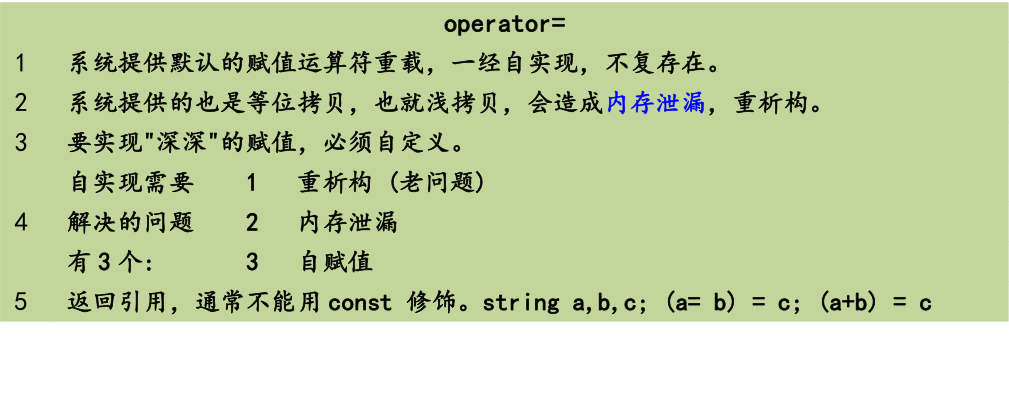展开全文• C++为了增强代码的可读性引入了运算符重载运算符重载是具有特殊函数名的函数，也具有其返回值类型，函数名字以及参数列表，其返回值类型与参数列表与普通的函数类似。 在C语言中，我们如果要实现两个数字相加，则...
日期类具体功能
1、获取每个月的天数
2、判断两个日期是否同一天
3、判断两个日期的先后
4、某日期在某天后的日期
5、某日期在某天前的日期
6、日期减日期所得天数
赋值运算符
C++为了增强代码的可读性引入了运算符重载，运算符重载是具有特殊函数名的函数，也具有其返回值类型，函数名字以及参数列表，其返回值类型与参数列表与普通的函数类似。
举个例子：如果我们想用赋值运算符实现两数相加，可以定义函数为int operator+=(int),最后返回*this，在测试时先定义一个常量（如int a），在调用该赋值运算符函数时，直接a+=b即可（b为想要加上的数字）。
函数名字为：关键字operator后面接需要重载的运算符符号。
函数原型：返回值类型 operator操作符(参数列表)。
注意：
1、不能通过连接其他符号来创建新的操作符：比如operator@ ；
2、重载操作符必须有一个类类型或者枚举类型的操作数用于内置类型的操作符，其含义不能改变，例如：内置的整型+，不能改变其含义；
3、作为类成员的重载函数时，其形参看起来比操作数数目少1成员函数的
操作符有一个默认的形参this，限定为第一个形参；
4、（*）（::）（sizeof）（?:）（.） 注意以上5个运算符不能重载。这个经常在笔试选择题中出现。
日期类代码实现
头文件
#pragma once
#include <iostream>
using namespace std;

class Date
{
public:
//打印日期
void Print();
//获取每个月的天数
int GetMonthDay(int year, int month);
//构造函数
Date(int year, int month, int day);
//拷贝构造函数
Date(const Date& d);

//判断两个日期是否同一天
bool operator==(Date& d);
bool operator!=(Date& d);

//判断两个日期的先后
bool operator>(Date& d);
bool operator<(Date& d);
bool operator<=(Date& d);
bool operator>=(Date& d);

//某日期在某天后的日期
Date operator+=(int day);
//某日期在某天后的日期
Date operator-=(int day);
//日期减日期所得天数
int operator-(Date& d);

private:
int _year;
int _month;
int _day;
};

源文件
#include"Date.h"

void Date::Print()
{
cout << _year << "-" << _month << "-" << _day << endl;
}
int Date::GetMonthDay(int year, int month)
{
static int dayArr = { 0,31,28,31,30,31,30,31,31,30,31,30,31 };
int days = dayArr[month];
if (month == 2 && ((year % 4 == 0 && year % 100 != 0) || (year % 400 == 0)))
{
days += 1;
}

return days;
}
Date::Date(int year, int month, int day)
{
_year = year;
_month = month;
_day = day;
if (!(year >= 0 && month > 0 && month < 13 && day > 0 && day <= GetMonthDay(year, month)))
{
cout << "日期非法" << endl;
}
}
Date::Date(const Date& d)
{
_year = d._year;
_month = d._month;
_day = d._day;
}

//判断两个日期是否同一天
bool Date::operator==(Date& d)
{
return d._year == _year && d._month == _month && d._day == _day;
}
bool Date::operator!=(Date& d)
{
return !(*this == d);
}
//判断两个日期的先后
bool Date::operator>(Date& d)
{
//return _year > d._year || _month > d._month || _day > d._day;
if (_year > d._year)
{
return true;
}
else if (_year == d._year)
{
if (_month > d._month)
return true;
}
else if (_year == d._year && _month == d._month)
{
if (_day > d._day)
return true;
}

return false;
}
bool Date::operator<(Date& d)
{
//return _year > d._year || _month > d._month || _day > d._day;
if (_year < d._year)
{
return true;
}
else if (_year == d._year)
{
if (_month < d._month)
return true;
}
else if (_year == d._year && _month == d._month)
{
if (_day < d._day)
return true;
}
return false;
}
bool Date::operator<=(Date& d)
{
return *this < d || *this == d;
}
bool Date::operator>=(Date& d)
{
return *this > d || *this == d;
}
//某日期在某天后的日期
Date Date::operator+=(int day)
{
_day += day;
while (_day > GetMonthDay(_year, _month))
{
_day -= GetMonthDay(_year, _month);
_month++;
if (_month == 13)
{
_year++;
_month = 1;
}
}

return *this;
}
//某日期在某天后的日期
Date Date::operator-=(int day)
{
_day -= day;
_month -= 1;
while (_day < 1)
{
_day += GetMonthDay(_year, _month);
_month--;
if (_month == 0)
{
_year--;
_month = 12;
}
}
_month += 1;
return *this;
}
//日期减日期所得天数
int Date::operator-(Date& d)
{
//默认*this为大的天数
Date max = *this, min = d;
int flag = 1;
//如果*this为小的天数，使flag=-1；最终相差天数也为负数
if (*this < d)
{
max = d;
min = *this;
flag = -1;
}
int n = 0;
while (min != max)
{
++n;
min += 1;
}
return n * flag;
}

测试源文件（main函数）
#include"Date.h"
int main()
{
Date d1(2000, 3, 30);
d1.Print();
cout << "该日期10000天后为";
d1 += 10000;
d1.Print();
Date d2(2021, 2, 5);
d2.Print();
cout << "该日期200天前为";
d2 -= 200;
d2.Print();
putchar('\n');

Date d3(2000, 3, 30);
Date d4(2021, 2, 5);
d3.Print();
d4.Print();
cout << "上面两天相差"<< d4 - d3 << "天" << endl;
}

运行截图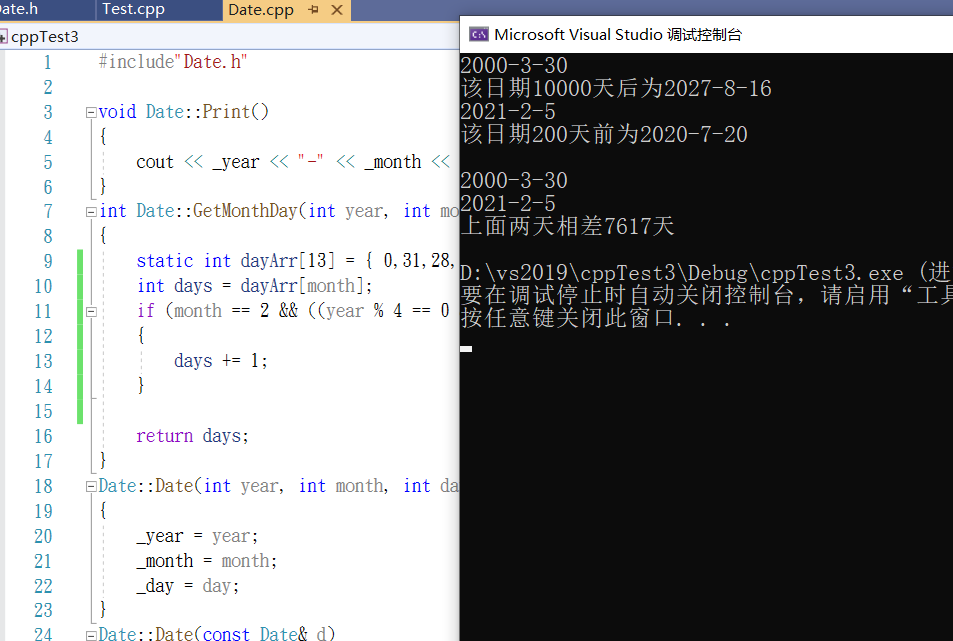展开全文c++
• 本文主要介绍C++中的赋值运算符重载函数（operator=）的相关知识。 1. 概述 1.1 why 首先介绍为什么要对赋值运算符“=”进行重载。某些情况下，当我们编写一个类的时候,，并不需要为该类重载“=”运算符，因为...
本文主要介绍C++中的赋值运算符重载函数（operator=）的相关知识。

1. 概述

1.1 why

首先介绍为什么要对赋值运算符“=”进行重载。某些情况下，当我们编写一个类的时候,，并不需要为该类重载“=”运算符，因为编译系统为每个类提供了默认的赋值运算符“=”，使用这个默认的赋值运算符操作类对象时，该运算符会把这个类的所有数据成员都进行一次赋值操作。例如有如下类：

class A
{
public:
int a;
int b;
int c;
};

那么对这个类的对象进行赋值时，使用默认的赋值运算符是没有问题的。示例代码（operator_test4.cpp）如下：

#include <iostream>

using namespace std;

class ClassA
{
public:
int a;
int b;
int c;
};

int main()
{
ClassA obj1;
obj1.a = 1;
obj1.b = 2;
obj1.c = 3;

ClassA obj2;
obj2 = obj1;

cout << "obj2.a is: " << obj2.a << endl;

return 0;
}



编译并执行上述代码，结果如下：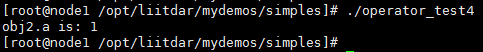从上述结果能够知道：通过使用系统默认的赋值运算符“=”，可以让对象obj2中的所有数据成员的值与对象obj1相同。这种情况下，编译系统提供的默认赋值运算符可以正常使用。

但是，在下面的示例中，使用编译系统默认提供的赋值运算符，就会出现问题了。示例代码（operator_test5.cpp）如下：

#include <iostream>
#include <string.h>

using namespace std;

class ClassA
{
public:
ClassA()
{

}

ClassA(const char* pszInputStr)
{
pszTestStr = new char[strlen(pszInputStr) + 1];
strncpy(pszTestStr, pszInputStr, strlen(pszInputStr) + 1);
}
virtual ~ClassA()
{
delete pszTestStr;
}
public:
char* pszTestStr;
};

int main()
{
ClassA obj1("liitdar");

ClassA obj2;
obj2 = obj1;

cout << "obj2.pszTestStr is: " << obj2.pszTestStr << endl;
cout << "addr(obj1.pszTestStr) is: " << &obj1.pszTestStr << endl;
cout << "addr(obj2.pszTestStr) is: " << &obj2.pszTestStr << endl;

return 0;
}


编译并运行上述代码，结果如下：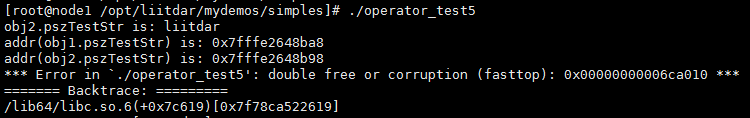上述错误信息说明：当obj1和obj2进行析构的时候，由于重复释放了一块内存，导致程序崩溃报错。在这种情况下，就需要我们重载赋值运算符“=”了。

2. 示例代码

2.1 示例代码1

我们修改一下前面出错的代码示例，现编写一个包含赋值运算符重载函数的类，代码（operator_test5.cpp）如下：

#include <iostream>
#include <string.h>

using namespace std;

class ClassA
{
public:
ClassA()
{

}
ClassA(const char* pszInputStr)
{
pszTestStr = new char[strlen(pszInputStr) + 1];
strncpy(pszTestStr, pszInputStr, strlen(pszInputStr) + 1);
}
virtual ~ClassA()
{
delete pszTestStr;
}
// 赋值运算符重载函数
ClassA& operator=(const ClassA& cls)
{
// 避免自赋值
if (this != &cls)
{
// 避免内存泄露
if (pszTestStr != NULL)
{
delete pszTestStr;
pszTestStr = NULL;
}

pszTestStr = new char[strlen(cls.pszTestStr) + 1];
strncpy(pszTestStr, cls.pszTestStr, strlen(cls.pszTestStr) + 1);
}

return *this;
}

public:
char* pszTestStr;
};

int main()
{
ClassA obj1("liitdar");

ClassA obj2;
obj2 = obj1;

cout << "obj2.pszTestStr is: " << obj2.pszTestStr << endl;
cout << "addr(obj1.pszTestStr) is: " << &obj1.pszTestStr << endl;
cout << "addr(obj2.pszTestStr) is: " << &obj2.pszTestStr << endl;

return 0;
}



编译并运行上述代码，结果如下：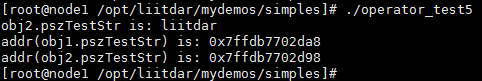通过上述结果能够看到，我们利用赋值运算符重载函数，解决了对象赋值的情况下，析构函数中过程中多次释放同一块内存的问题。

对于上述代码，有以下几点需要说明：

当为一个类的对象赋值（可以用本类对象为其赋值，也可以用其它类型的值为其赋值）时，该对象（如本例的obj2）会调用该类的赋值运算符重载函数，进行具体的赋值操作。如上述代码中的“obj2 = obj1;”语句，用obj1为obj2赋值，则会由obj2调用ClassA类的赋值运算符重载函数。
语句“ClassA obj2;
obj2 = obj1;“
和语句“ClassA obj2 = obj1;”在调用函数上是有区别的：前者第一句是对象obj2的声明及定义，调用类ClassA的无参构造函数，所以“obj2 = obj1;”一句是在对象obj2已经存在的情况下，用obj1来为obj2赋值，调用的是赋值运算符重载函数；而后者，是用obj1来初始化obj2，调用的是拷贝构造函数。关于拷贝构造函数的语句样式为“ClassA(const ClassA& cls)”，关于拷贝构造函数的内容，此处不进行详述。
当程序没有显式地提供一个以“本类或本类的引用”为参数的赋值运算符重载函数时，编译器会自动生成一个默认的赋值运算符重载函数。

2.2 示例代码2

示例代码（operator_test6.cpp）如下：

#include<iostream>
#include<string>

using namespace std;

class Data
{
private:
int data;

public:
// 构造函数
Data()
{
};
// 构造函数
Data(int _data):data(_data)
{
cout << "This is constructor" << endl;
}
// 赋值运算符重载函数
Data& operator=(const int _data)
{
cout << "This is operator=(int _data)" << endl;
data = _data;

return *this;
}
};

int main()
{
// 调用构造函数
Data data1(1);
Data data2, data3;
// 调用赋值运算符重载函数
data2 = 1;
// 调用默认的赋值运算符重载函数
data3 = data2;

return 0;
}


编译并执行上述代码，结果如下：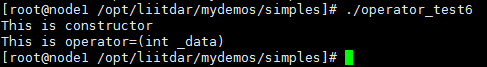上述结果说明：“data2 = 1;”语句调用了我们提供的以int型参数（而非本类或本类的引用）为形参的赋值运算符重载函数，而“data3 = data2;”的成功执行，说明该语句调用了编译器提供的默认的赋值运算符重载函数。

如果将上述代码中赋值运算符重载函数去掉，重新编译执行，结果如下：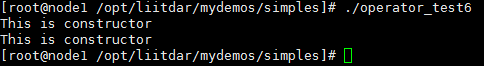上述结果说明，当用一个非类A的值（如上面的int类型值）为类A的对象赋值时：

如果检测到构造函数和赋值运算符重载函数同时存在，则会调用赋值运算符重载函数；
如果检测到的构造函数，就会调用这个构造函数。
3. 总结

综合上述示例内容，我们可以知道针对以下情况，需要显式地提供赋值运算符重载函数（即自定义赋值运算符重载函数）：

用非类A类型的值为类A的对象赋值时（当然，这种情况下我们可以不提供相应的赋值运算符重载函数，而只提供相应的构造函数，如更改后的示例代码2）。
当用类A类型的值为类A的对象赋值，且类A的数据成员中含有指针的情况下，必须显式提供赋值运算符重载函数（如示例代码1）。

展开全文C++ operator
• 在我之前的文章中，介绍了算术运算符重载的基本使用，现在我将继续介绍关系运算符重载和赋值运算符重载。 还没看过的朋友可以点击链接前去看一下：C++ 运算符重载 ...其实他们之间的用法都是类似的，没有什么区别，都是...c++
• 12.5.4 赋值运算符重载 我们再创建一个类对象后，有时会有这样的操作。 Person p1("张三", 18); person p2; p2 = p1; 注意看第三行代码，使用了赋值运算符，按理说没有重载前赋值运算符应该不能操作自定义数据...c++
• C++赋值运算符重载 赋值运算符重载和拷贝构造函数的作用类似，都是将类对象进行复制。 需要注意的点也是一样，就是堆区重复释放问题 这个问题的诱因就是，编译器默认的拷贝是浅拷贝，而浅拷贝会出现重复释放内存的...c++
• 文章目录前言一、赋值运算符重载函数是什么？二、细谈赋值运算符重载函数2.1 参数列表2.2 返回值2.3调用时机二、赋值运算符重载函数练习 前言 在介绍赋值运算符重载之前，我们先看一段代码： class Complex //定义...c++...